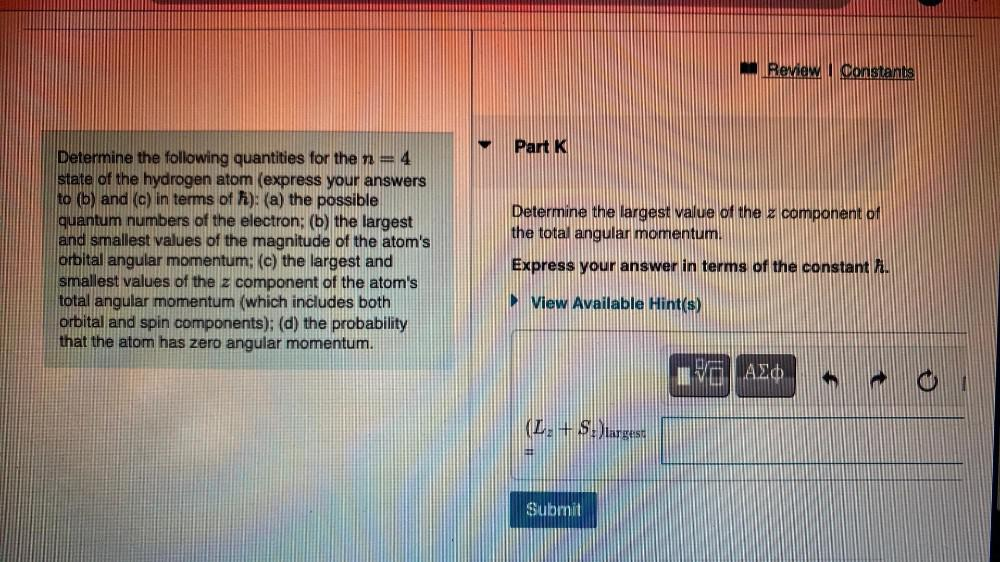Question:

# Review | Constants Part K Determine the largest value of the x component of the total angular momentum. Determine the followingReview | Constants Part K Determine the largest value of the x component of the total angular momentum. Determine the following quantities for the n= 4 state of the hydrogen atom (express your answers to (b) and (c) in terms of H): (a) the possible quantum numbers of the electron: (b) the largest and smallest values of the magnitude of the atom's orbital angular momentum; (c) the largest and smallest values of the z component of the atom's total angular momentum (which includes both orbital and spin components); (d) the probability that the atom has zero angular momentum. Express your answer in terms of the constant . View Available Hint(s) VO AZO 1 61 (L+SH)largest